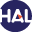Home page > 13. Volume 8 (2012) > Rational Approximation in the sense of Kato for transport semigroups

# Rational Approximation in the sense of Kato for transport semigroups

Monday 14 May 2012,

Abstract: In this paper we mix the rational approximation procedure, which is a time approximation with approximation in the sense of Kato, which is a space approximation for linear transport equation. In 1970, H. J. Hejtmanek gave such a procedure for approximation of the linear transport equation and he proved the convergence only for explicit Euler scheme. We extend this procedure to explicit and implicit Euler, Crank-Nicolson and Predictor-Corrector schemes which have the rate 1,2 and 3 in the sense of rational approximation. Finally, we construct the numerical illustration for justifying the above rate of convergence.

Key words. Acceptable rational function. Euler’s explicit and implicit algorithms. Crank- Nicolson scheme. Predictor-Corrector algorithm. Rate of convergence. Free, absorption, production transport semigroups

Paper presented by : Professor Serge NicaiseRational Approximation in the sense of Kato for transport semigroupsVoir sur HAL : https://hal.archives-ouvertes.fr/hal-01121332